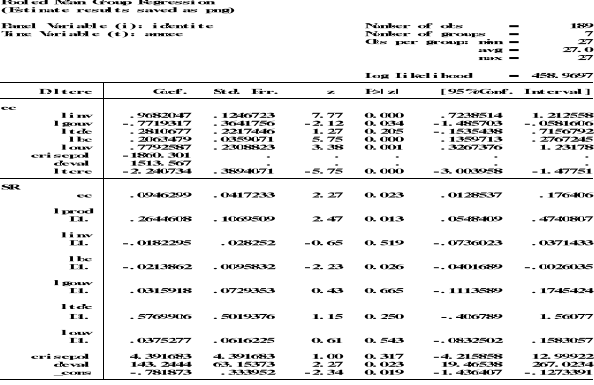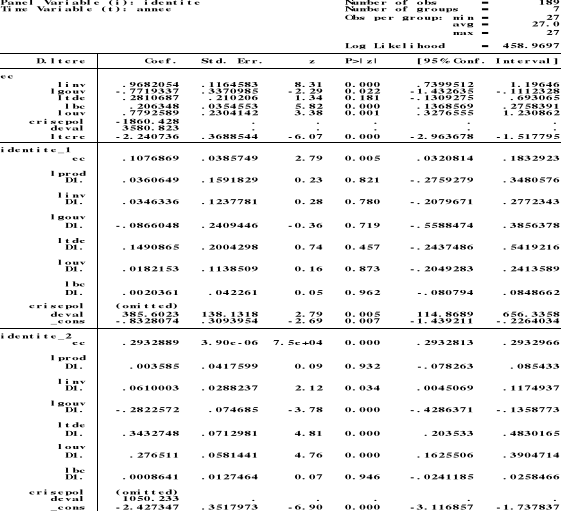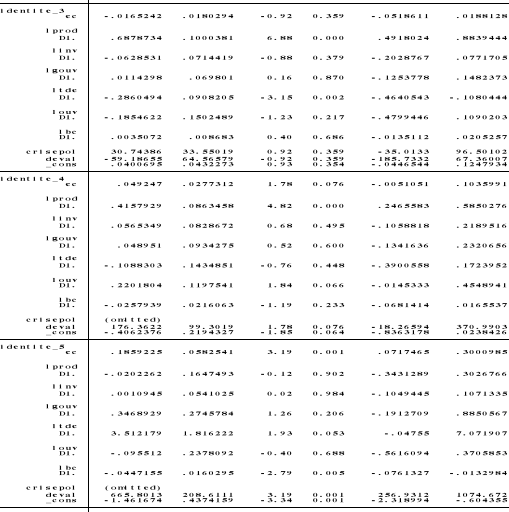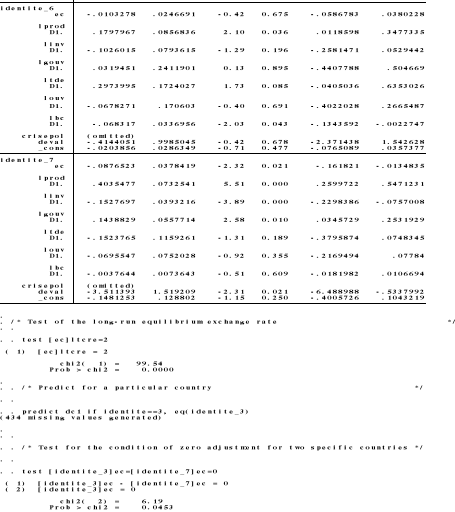WOW !! MUCH LOVE ! SO WORLD PEACE !
Fond bitcoin pour l'amélioration du site: 1memzGeKS7CB3ECNkzSn2qHwxU6NZoJ8o
Dogecoin (tips/pourboires): DCLoo9Dd4qECqpMLurdgGnaoqbftj16NvpRechercher sur le site:

# Fondamentaux du taux de change réel et mésalignements du franc CFA dans l'UEMOA

( Télécharger le fichier original )
Université de Lomé Togo - Master en économie internationale 2012

# Annexes

ANNEXE 1 : Résultats des tests de racine unitaire en panel sous Eviews 7

 Panel unit root test: Summary ` ` Series: D(LBC) ` ` ` ` Date: 12/10/11 Time: 10:20 ` ` Sample: 1984 2010 ` ` ` ` Exogenous variables: Individual effects, individual linear trends User-specified lags: 1 ` ` ` ` Newey-West automatic bandwidth selection and Bartlett kernel Balanced observations for each test ` ` ` ` ` ` ` ` ` ` ` ` ` ` ` ` ` ` ` ` ` ` ` ` ` ` ` ` Cross- ` ` Method Statistic Prob.** sections Obs Null: Unit root (assumes common unit root process) Levin, Lin & Chu t* -6.17890 0.0000 7 168 Breitung t-stat -3.82736 0.0001 7 161 ` ` ` ` ` ` ` ` ` ` Null: Unit root (assumes individual unit root process) Im, Pesaran and Shin W-stat -7.10548 0.0000 7 168 ADF - Fisher Chi-square 70.6214 0.0000 7 168 PP - Fisher Chi-square 432.809 0.0000 7 175 ` ` ` ` ` ` ` ` ` ` ` ` ` ` ` ` ` ` ` ` ** Probabilities for Fisher tests are computed using an asymptotic Chi -square distribution. All other tests assume asymptotic normality. Panel unit root test: Summary ` ` Series: D(LGOUV) ` ` ` ` Date: 12/10/11 Time: 10:12 ` ` Sample: 1984 2010 ` ` ` ` Exogenous variables: None ` ` ` ` User-specified lags: 1 ` ` ` ` Newey-West automatic bandwidth selection and Bartlett kernel Balanced observations for each test ` ` ` ` ` ` ` ` ` ` ` ` ` ` ` ` ` ` ` ` ` ` ` ` ` ` ` ` Cross- ` ` Method Statistic Prob.** Sections Obs Null: Unit root (assumes common unit root process) Levin, Lin & Chu t* -11.2131 0.0000 7 168 ` ` ` ` ` ` ` ` ` ` Null: Unit root (assumes individual unit root process) ADF - Fisher Chi-square 124.757 0.0000 7 168 PP - Fisher Chi-square 264.459 0.0000 7 175 ` ` ` ` ` ` ` ` ` ` ` ` ` ` ` ` ` ` ` ` ** Probabilities for Fisher tests are computed using an asymptotic Chi -square distribution. All other tests assume asymptotic normality. Panel unit root test: Summary ` ` Series: D(LINV) ` ` ` ` Date: 12/10/11 Time: 10:05 ` ` Sample: 1984 2010 ` ` ` ` Exogenous variables: None ` ` ` ` User-specified lags: 1 ` ` ` ` Newey-West automatic bandwidth selection and Bartlett kernel Balanced observations for each test ` ` ` ` ` ` ` ` ` ` ` ` ` ` ` ` ` ` ` ` ` ` ` ` ` ` ` ` Cross- ` ` Method Statistic Prob.** sections Obs Null: Unit root (assumes common unit root process) Levin, Lin & Chu t* -11.0383 0.0000 7 168 ` ` ` ` ` ` ` ` ` ` Null: Unit root (assumes individual unit root process) ADF - Fisher Chi-square 121.700 0.0000 7 168 PP - Fisher Chi-square 276.858 0.0000 7 175 ` ` ` ` ` ` ` ` ` ` ` ` ` ` ` ` ` ` ` ` ** Probabilities for Fisher tests are computed using an asymptotic Chi -square distribution. All other tests assume asymptotic normality. Panel unit root test: Summary ` ` Series: D(LOUV) ` ` ` ` Date: 12/10/11 Time: 09:57 ` ` Sample: 1984 2010 ` ` ` ` Exogenous variables: Individual effects, individual linear trends User-specified lags: 1 ` ` ` ` Newey-West automatic bandwidth selection and Bartlett kernel Balanced observations for each test ` ` ` ` ` ` ` ` ` ` ` ` ` ` ` ` ` ` ` ` ` ` ` ` ` ` ` ` Cross- ` ` Method Statistic Prob.** sections Obs Null: Unit root (assumes common unit root process) Levin, Lin & Chu t* -6.78052 0.0000 7 168 Breitung t-stat -4.48796 0.0000 7 161 ` ` ` ` ` ` ` ` ` ` Null: Unit root (assumes individual unit root process) Im, Pesaran and Shin W-stat -6.60651 0.0000 7 168 ADF - Fisher Chi-square 65.1881 0.0000 7 168 PP - Fisher Chi-square 225.300 0.0000 7 175 ` ` ` ` ` ` ` ` ` ` ` ` ` ` ` ` ` ` ` ` ** Probabilities for Fisher tests are computed using an asymptotic Chi -square distribution. All other tests assume asymptotic normality. Panel unit root test: Summary ` ` Series: LPROD ` ` ` ` Date: 12/10/11 Time: 09:45 ` ` Sample: 1984 2010 ` ` ` ` Exogenous variables: Individual effects, individual linear trends User-specified lags: 1 ` ` ` ` Newey-West automatic bandwidth selection and Bartlett kernel Balanced observations for each test ` ` ` ` ` ` ` ` ` ` ` ` ` ` ` ` ` ` ` ` ` ` ` ` ` ` ` ` Cross- ` ` Method Statistic Prob.** sections Obs Null: Unit root (assumes common unit root process) Levin, Lin & Chu t* -4.64167 0.0000 7 175 Breitung t-stat -7.62524 0.0000 7 168 ` ` ` ` ` ` ` ` ` ` Null: Unit root (assumes individual unit root process) Im, Pesaran and Shin W-stat -3.46625 0.0003 7 175 ADF - Fisher Chi-square 34.9128 0.0015 7 175 PP - Fisher Chi-square 63.8712 0.0000 7 182 ` ` ` ` ` ` ` ` ` ` ` ` ` ` ` ` ` ` ` ` ** Probabilities for Fisher tests are computed using an asymptotic Chi -square distribution. All other tests assume asymptotic normality. Panel unit root test: Summary ` ` Series: D(LTCRE) ` ` ` ` Date: 12/10/11 Time: 09:34 ` ` Sample: 1984 2010 ` ` ` ` Exogenous variables: None ` ` ` ` User-specified lags: 1 ` ` ` ` Newey-West automatic bandwidth selection and Bartlett kernel Balanced observations for each test ` ` ` ` ` ` ` ` ` ` ` ` ` ` ` ` ` ` ` ` ` ` ` ` ` ` ` ` ` ` ` ` Method Statistic Prob.** sections Obs Null: Unit root (assumes common unit root process) Levin, Lin & Chu t* -9.09716 0.0000 7 168 ` ` ` ` ` ` ` ` ` ` Null: Unit root (assumes individual unit root process) ADF - Fisher Chi-square 102.907 0.0000 7 168 PP - Fisher Chi-square 166.956 0.0000 7 175 ` ` ` ` ` ` ` ` ` ` ` ` ` ` ` ` ` ` ` ` ** Probabilities for Fisher tests are computed using an asymptotic Chi -square distribution. All other tests assume asymptotic normality. Panel unit root test: Summary Series: D(LTCRE)Date: 12/10/11 Time: 11:44Sample: 1984 2010Exogenous variables: Individual effects, individual linear trendsUser-specified lags: 1Newey-West automatic bandwidth selection and Bartlett kernelBalanced observations for each test Cross-MethodStatisticProb.**sectionsObsNull: Unit root (assumes common unit root process) Levin, Lin & Chu t*-4.01081 0.0000 6 144Breitung t-stat-4.57734 0.0000 6 138Null: Unit root (assumes individual unit root process) Im, Pesaran and Shin W-stat -4.93073 0.0000 6 144ADF - Fisher Chi-square 45.3630 0.0000 6 144PP - Fisher Chi-square 103.060 0.0000 6 150** Probabilities for Fisher tests are computed using an asymptotic Chi        -square distribution. All other tests assume asymptotic normality. ` `

ANNEXE 2: Résultats du test du cointégration des séries

 Kao Residual Cointegration Test ` ` Series: LTCRE LTDE LPROD LOUV LINV LGOUV LBC ` ` Date: 12/10/11 Time: 10:27 ` ` ` ` Sample: 1984 2010 ` ` ` ` Included observations: 189 ` ` ` ` Null Hypothesis: No cointegration ` ` Trend assumption: No deterministic trend ` ` User-specified lag length: 1 ` ` ` ` Newey-West automatic bandwidth selection and Bartlett kernel ` ` ` ` ` ` ` ` ` ` ` ` ` ` ` ` ` ` ` ` ` ` ` ` ` ` t-Statistic Prob. ADF ` ` ` ` -3.075414 0.0011 ` ` ` ` ` ` ` ` ` ` ` ` ` ` ` ` ` ` ` ` Residual variance 0.001395 ` ` HAC variance ` ` 0.000934 ` ` ` ` ` ` ` ` ` ` ` ` ` ` ` ` ` ` ` ` ` ` ` ` ` ` ` ` ` ` ` ` ` ` ` ` ` ` ` ` ` ` ` ` ` ` ` ` ` ` ` `

ANNEXE 3 : Résultats des estimations de la relation entre le taux de change réel et ses fondamentaux à l'aide du logiciel STATA 11